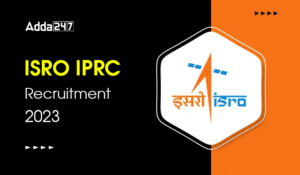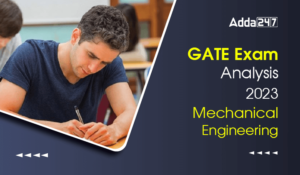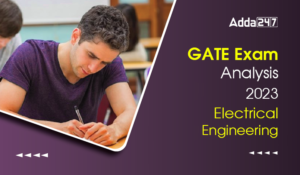Engineering Jobs   »   Mechincal Engineering quizs   »   UPPSC Lecturer Recruitment 2021, UPPSC Lecturer...

# UPPSC Lec’21 ME: Daily Practices Quiz. 10-Dec-2021

Quiz: Mechanical Engineering
Exam: UPPSC AE/Lecturer
Topic: RAC

Each question carries 3 marks
Negative marking: 1 mark
Time: 8 Minutes

Q1. A heat pump working on reversed Carnot cycle has a COP of 7. What is the ratio of maximum to minimum absolute temperatures?
(a) 7/8
(b) 1/7
(c) 7/6
(d) 6/7

Q2. A Carnot refrigerator has a COP of 6. What is the COP of heat pump?
(a) 7/6
(b) 5
(c) 7
(d) 1/6

Q3. In case of vapour-compression refrigeration system, the enthalpies at various points in the cycle are:
1. Enthalpy at inlet to the compressor (saturated vapour), h₁ = 280kJ/kg
2. Enthalpy at outlet of the compressor (after isentropic compression), h₂ = 300 kJ/kg
3. Enthalpy at exit of the condenser (saturated liquid), h_3 = 180 kJ/kg
What is the COP of the plant?
(a) 3
(b) 4
(c) 5
(d) 6

Q4. Ammonia used as refrigerant is non-corrosive to
(a) iron and steel
(b) copper and copper alloys
(c) Both (a) and (b)
(d) Neither (a) nor (b)

Q5. In a VCR plant, the refrigerant leaves the compressor and evaporator with enthalpy of 205 kJ/kg and 177 kJ/kg respectively. Enthalpy of the refrigerant leaving the condenser is 105 kJ/kg. If the mass flow rate of the refrigerant is 0.2 kg/s, the refrigeration effect will be
(a) 12.2 kW
(b) 14.4 kW
(c) 16.4 kW
(d) 20.2 kW

Q6. The expression for COP of reversed Brayton cycle is (r_P is the pressure ratio)
(a) 1/(〖r_p〗^((γ-1)/γ)-1)
(b) 1/(〖r_p〗^γ-1)
(c) 1/〖r_p〗^((γ-1)/γ)
(d) 1/(〖r_p〗^((γ-1)/γ)+1)

Solutions

S1. Ans.(c)
Sol.

As, we know that,
〖(COP)〗_HP= ( T_H )/(T_H – T_L )
7 = ( 1 )/(1 -T_L/T_H )
1/7=1-T_L/T_H
T_L/T_H =1-1/7=6/7
T_H/T_L =7/6

S2. Ans.(c)
Sol.
As we know that, COP of refrigerator is
〖(COP)〗_R= ( T_L )/(T_H – T_L )
〖(COP)〗_R=6

COP of the heat pump is
(COP)_HP=1+(COP)_R
〖(COP)〗_HP=1+6=7
S3. Ans. (c)
Sol. Enthalpies at various points are
h₁ = 280kJ/kg
h₂ = 300 kJ/kg
h_3 = 180 kJ/kg
As, we know that COP of VCRS is
COP = (h_1 – h_3)/(h_2 – h_1 )=(280-180)/(300-280)=100/20 = 5

S4. Ans.(a)
Sol.
The refrigerant ammonia is corrosive to copper and copper alloys but it is non corrosive to iron and steel.

S5. Ans.(b)
Sol.
Refrigerant Effects = Enthalpy at outlet of evaporator – Enthalpy at outlet of condenser
RE = 177 – 105 = 72 kJ/kg
RE = 72 × 0.2 = 14.4 kW

S6. Ans (a)
Sol.
(〖COP)〗_(Reversed Brayton)=1/(〖r_p〗^((γ-1)/γ)-1)
Here, r_P is the pressure ratio.

Sharing is caring!

•GATE 2023 Admit Card Out, Download Link ...
•GATE Exam Analysis 2023 Computer Science...
•GATE 2023 Memory Based Question Paper of...
•ISRO IPRC Recruitment 2023 Notification ...
•GATE Exam Analysis 2023 Mechanical Engin...
•GATE Exam Analysis 2023 Electrical Engin...
•NIT Warangal Recruitment 2023 Notificati...